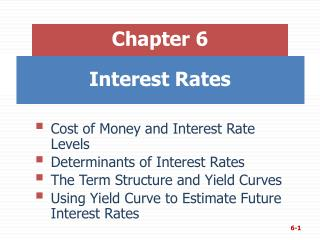DownloadDownload PresentationInterest Rates

# Interest Rates

Download Presentation## Interest Rates

- - - - - - - - - - - - - - - - - - - - - - - - - - - E N D - - - - - - - - - - - - - - - - - - - - - - - - - - -
##### Presentation Transcript

1. Chapter 6 • Cost of Money and Interest Rate Levels • Determinants of Interest Rates • The Term Structure and Yield Curves • Using Yield Curve to Estimate Future Interest Rates Interest Rates

2. What four factors affect the level of interest rates? • Production opportunities • Time preferences for consumption • Risk • Expected inflation

3. “Nominal” vs. “Real” Rates r = represents any nominal rate r* = represents the “real” risk-free rate of interest. Like a T-bill rate, if there was no inflation. Typically ranges from 1% to 5% per year. rRF = represents the rate of interest on Treasury securities.

4. Determinants of Interest Rates r = r* + IP + DRP + LP + MRP r = required return on a debt security r* = real risk-free rate of interest IP = inflation premium DRP = default risk premium LP = liquidity premium MRP = maturity risk premium

6. Yield Curve and the Term Structure of Interest Rates • Term structure – relationship between interest rates (or yields) and maturities. • The yield curve is a graph of the term structure. • The October 2008 Treasury yield curve is shown at the right.

7. Step 1 – Find the average expected inflation rate over Years 1 to N: Constructing the Yield Curve: Inflation

8. Constructing the Yield Curve: Inflation Assume inflation is expected to be 5% next year, 6% the following year, and 8% thereafter. Must earn these IPs to break even vs. inflation; these IPs would permit you to earn r* (before taxes).

9. Constructing the Yield Curve: Maturity Risk • Step 2 – Find the appropriate maturity risk premium (MRP). For this example, the following equation will be used to find a security’s appropriate maturity risk premium. MRPt = 0.1% (t – 1)

10. Constructing the Yield Curve: Maturity Risk Using the given equation: Notice that since the equation is linear, the maturity risk premium is increasing as the time to maturity increases, as it should be.

11. Add the IPs and MRPs to r* to Find the Appropriate Nominal Rates Step 3 – Adding the premiums to r*. rRF, t = r* + IPt + MRPt Assume r* = 3%,

12. Hypothetical Yield Curve • An upward sloping yield curve. • Upward slope due to an increase in expected inflation and increasing maturity risk premium. Interest Rate (%) 15 Maturity risk premium 10 Inflation premium 5 Real risk-free rate Years to Maturity 0 1 10 20

13. Relationship Between Treasury Yield Curve and Yield Curves for Corporate Issues • Corporate yield curves are higher than that of Treasury securities, though not necessarily parallel to the Treasury curve. • The spread between corporate and Treasury yield curves widens as the corporate bond rating decreases.

14. BB-Rated AAA-Rated Illustrating the Relationship Between Corporate and Treasury Yield Curves Interest Rate (%) 15 10 Treasury Yield Curve 6.0% 5.9% 5 5.2% Years to Maturity 0 0 1 5 10 15 20

15. Pure Expectations Hypothesis • The PEH contends that the shape of the yield curve depends on investor’s expectations about future interest rates. • If interest rates are expected to increase, L-T rates will be higher than S-T rates, and vice-versa. Thus, the yield curve can slope up, down, or even bow.

16. Assumptions of the PEH • Assumes that the maturity risk premium for Treasury securities is zero. • Long-term rates are an average of current and future short-term rates. • If PEH is correct, you can use the yield curve to “back out” expected future interest rates.

17. An Example:Observed Treasury Rates and the PEH If PEH holds, what does the market expect will be the interest rate on one-year securities, one year from now? Three-year securities, two years from now?

18. One-Year Forward Rate (1.062)2 = (1.060) (1 + X) 1.12784/1.060 = (1 + X) 6.4004% = X 6.0% x% 0 1 2 6.2% • PEH says that one-year securities will yield 6.4004%, one year from now. • Notice, if an arithmetic average is used, the answer is still very close. Solve: 6.2% = (6.0% + X)/2, and the result will be 6.4%.

19. Three-Year Security, Two Years from Now (1.065)5 = (1.062)2 (1 + X)3 1.37009/1.12784 = (1 + X)3 6.7005% = X 6.2% x% 0 1 2 3 4 5 6.5% • PEH says that three-year securities will yield 6.7005%, two years from now.

20. Conclusions about PEH • Some would argue that the MRP ≠ 0, and hence the PEH is incorrect. • Most evidence supports the general view that lenders prefer S-T securities, and view L-T securities as riskier. • Thus, investors demand a premium to persuade them to hold L-T securities (i.e., MRP > 0).

21. Macroeconomic Factors That Influence Interest Rate Levels • Federal reserve policy • Federal budget deficits or surpluses • International factors • Level of business activity